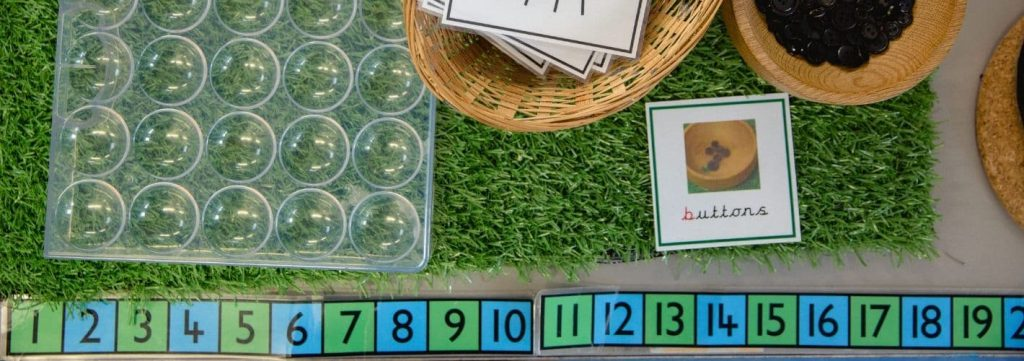Mathematics‘The only way to learn mathematics is to do mathematics.’

Paul Halmos

National Curriculum 2014

In mathematics, pupils will have the opportunity to:

• become fluent in the fundamentals of mathematics, through frequent practice with increasingly difficult problems over time
• develop their understanding of mathematical concepts to be able to recall and apply their knowledge rapidly and accurately to problems
• reason mathematically by following a line of enquiry, connecting relationships and developing an argument, justification or proof using mathematical language
• solve problems by applying their mathematics to a variety of routine and non-routine problems with increasing sophistication, including breaking down problems into a series of simpler steps and persevering when seeking solutions.

Inspired by Chris Quigley Ltd

Pupils will be able to develop their skills as a mathematician by:

• understanding mathematical concepts
• developing a range of skills and using and applying these in mathematics
• understanding number facts and the number system
• practising skills as a problem solver and developing an ordered approach to solving problems in a wide range of contexts
• being an independent thinker who perseveres when faced with challenges and will have a go!
• learning from their mistakes and seeing these as a positive learning experience
• reasoning and summarising, make sense of their findings.